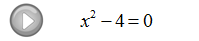## Algebra

Showing posts with label zero property. Show all posts
Showing posts with label zero property. Show all posts

### Solving Equations by Factoring

Previously we have learned how to solve linear equations, now we will outline a technique used to solve factorable quadratic equations that look like

In addition, we will revisit function notation and apply the techniques in this section to quadratic functions.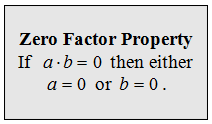The above zero factor property is the key to solving quadratic equations by factoring. So far we have been solving linear equations, which usually had only one solution. We will see that quadratic equations have up to two solutions.

Solve:

Step 1: Obtain zero on one side and then factor.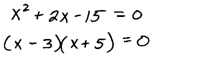Step 2:  Set each factor equal to zero.Step 3: Solve each of the resulting equations.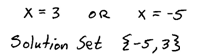This technique requires the zero factor property to work so make sure the quadratic is set equal to zero before factoring in step 1.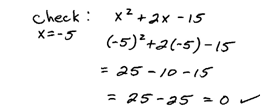Tip: You can always see if you solved correctly by checking your answers. On an exam it is useful to know if got the correct solutions or not.

Solve.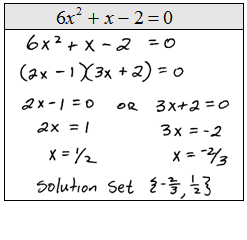When solving quadratic equations by factoring, the first step is to put the equation in standard form ax^2 + bx + c = 0, equal to zero.

Solve:Obtain standard form and then factor.Set each factor equal to zero and solve.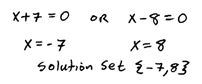Check.Important: We must have zero on one side of our equation for this technique to work.

Solve.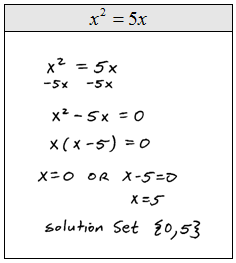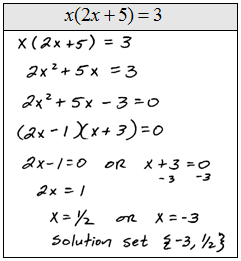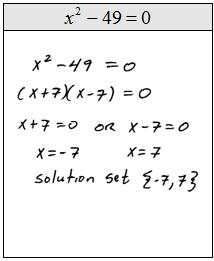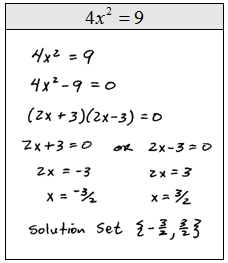You can clear fractions from any equation by multiplying both sides by the LCD.

Solve:Multiply both sides by the LCD 6 here to clear the fractions.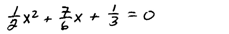Solve.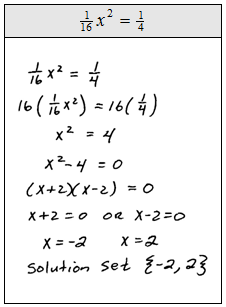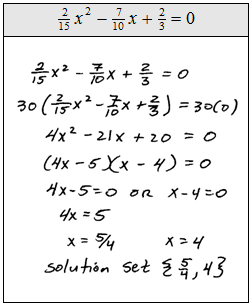Work the entire process in reverse to find equations given the solutions.

Find a quadratic equation with given solution set.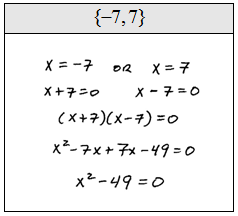Remember that the notation y = f(x) reads, "y equals of x."Evaluate the given function.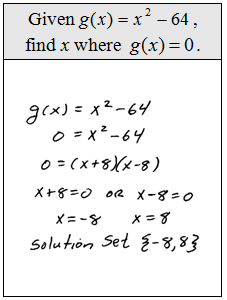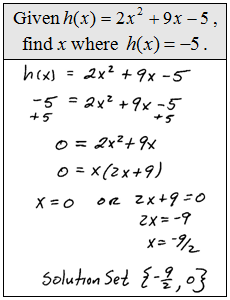Given the graph of the quadratic function, find the x- and y- intercepts.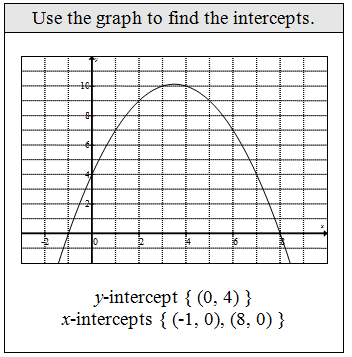Video Examples on YouTubeSolve by Factoring PlayList